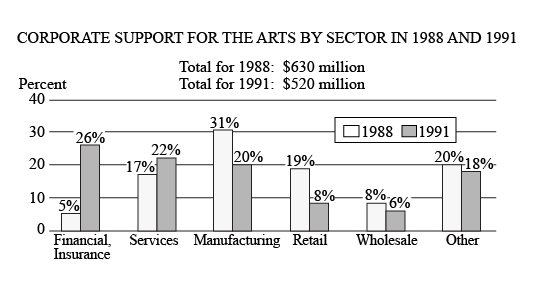# From 1988 to 1991, which corporate sector decreased its supportFrom $1988$ to $1991$, which corporate sector decreased its support for the arts by the greatest dollar amount?

1. Services
2. Manufacturing
3. Retail
4. Wholesale
5. Other

So, you were trying to be a good test taker and practice for the GRE with PowerPrep online. Buuuut then you had some questions about the quant section—specifically question 15 of the second Quantitative section of Practice Test 1. Those questions testing our knowledge of Graphical Methods for Describing Data can be kind of tricky, but never fear, PrepScholar has got your back!

## Survey the Question

Let’s search the problem for clues as to what it will be testing, as this will help shift our minds to think about what type of math knowledge we’ll use to solve this question. Pay attention to any words that sound math-specific and anything special about what the numbers look like, and mark them on our paper.

Oh, a clustered bar graph! This should be fun. It’ll definitely draw on what we’ve learned about Graphical Methods for Describing Data. Let’s keep what we’ve learned about this skill at the tip of our minds as we approach this question.

## What Do We Know?

Let’s carefully read through the question and make a list of the things that we know.

1. We have a clustered bar graph
2. We want to know which sector decreased its contributions the most going from $1988$ to $1991$

## Develop a Plan

We want to know which of the six sectors decreased its contribution the most going from $1988$ to $1991$. We can see that the Financial and Services sectors both have increased percentages going from $1988$ to $1991$, so doesn’t look like it’ll be either of those sectors. Looking at the other four sectors, the Wholesale and Other sectors barely decreased their percentages at all, each only going down $2$ percentage points, so doesn’t look like either of them will be the right answer. The Manufacturing and Retail sectors had substantial drops in the percentage contributions. Let’s calculate how much each lost going from $1988$ to $1991$ for the Manufacturing and Retail sectors.

## Solve the Question

To calculate the drop in contributions, we can just take the difference between the product of the total for that year and the percentage the sector contributed that year, remembering that we need to divide percentages by $100$ before using them in equations:

$$\Dollar \Drop \Off = {\Percent \in 1988}/100·\Total \for 1988 – {\Percent \in 1991}/100·\Total \for 1991$$

Let’s calculate this for the Manufacturing sector now.

 $\Manufacturing \Dollar \Drop \Off$ $=$ ${\Percent \in 1988}/100·\Total \for 1988 – {\Percent \in 1991}/100·\Total \for 1991$   $\Manufacturing \Dollar \Drop \Off$ $=$ $31/100·\$630 \million – 20/100·\$520 \million$   $\Manufacturing \Dollar \Drop \Off$ $=$ $\$195.3 \million – \$104 \million$   $\Manufacturing \Dollar \Drop \Off$ $=$ $\$91.3 \million$Let’s calculate this for the Retail sector now. $\Retail \Dollar \Drop \Off={\Percent \in 1988}/100·\Total \for 1988 – {\Percent \in 1991}/100·\Total \for 1991  \Retail \Dollar \Drop \Off=19/100·\$630 \million – 8/100·\$520 \million  \Retail \Dollar \Drop \Off=\$119.7 \million – \$41.6 \million  \Retail \Dollar \Drop \Off=\$78.1 \million$

While the Retail sector decreased by only $\$78.1$million, the Manufacturing sector decreased by$\$91.3$ million. Since the Manufacturing sector decreased the most, The correct answer is B, Manufacturing.

## What Did We Learn

Strangely, we might have noticed that both the Manufacturing and Retail sectors each decreased by $11$ percentage points going from $1988$ to $1991$, yet they had different decreases as measured in dollar amounts. Percentages can be strange things. Let’s make sure we’re careful to fully do calculations with them for that very reason.

Want more expert GRE prep? Sign up for the five-day free trial of our PrepScholar GRE Online Prep Program to access your personalized study plan with 90 interactive lessons and over 1600 GRE questions.